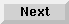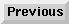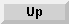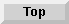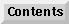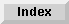2.2 Iteration control

# 2.2.1 Using multiple iteration clauses

If you use multiple iteration clauses to control iteration, variable initialization and stepping occur sequentially by default. You can use the`and` construct to connect two or more iteration clauses when sequential binding and stepping are not necessary. The iteration behavior of clauses joined by`and` is analogous to the behavior of the Common Lisp macro`do` with respect to`do*`.

In the following example, the variable`x` is stepped before`y` is stepped; thus, the value of`y` reflects the updated value of`x`:

```> (loop for x from 1 to 10
for y = nil then x
collect (list x y))
((1 NIL) (2 2) (3 3) (4 4) (5 5) (6 6) (7 7) (8 8) (9 9) (10 10))

```
In this example,`x` and`y` are stepped in parallel:

```> (loop for x from 1 to 10
and
for y = nil then x
collect (list x y))
((1 NIL) (2 1) (3 2) (4 3) (5 4) (6 5) (7 6) (8 7) (9 8) (10 9))

```

The Loop Facility - 9 SEP 1996Generated with Harlequin WebMaker